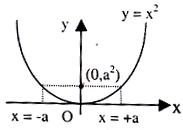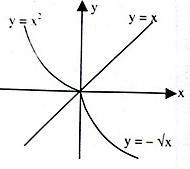×#### Thank you for registering.

One of our academic counsellors will contact you within 1 working day.

Click to Chat

1800-1023-196

+91-120-4616500

CART 0

• 0

MY CART (5)

Use Coupon: CART20 and get 20% off on all online Study Material

ITEM
DETAILS
MRP
DISCOUNT
FINAL PRICE
Total Price: Rs.

There are no items in this cart.
Continue Shopping```Invertible FunctionLet us define a function y = f(x): X → Y. If we define a function g(y) such that x = g(y) then g is said to be the inverse function of 'f'.

Think: If f is many-to-one, g : Y → X will not satisfy the definition of a function.

So to define the inverse of a function, it must be one-one.

Further if f : X → Y is into then there must be a point in Y for which  there is no x. This again violates the definition of function for 'g' (In fact when f is one tone and onto then 'g' can be defined from range of f to domain of i.e. g : f(X) → X.

Hence, the inverse of a function can be defined within the same sets   for x and Y only when it is one-one and onto or Bijective.

Note: A monotonic function i.e. bijection function is always invertible.

Illustration: Let f : R → R be defined as

y = f(x) = x2. Is it invertible?

Solution:

No it is not invertible because this is a many one into function

This is many-one because for x = + a, y = a2, this is into because y does not take the negative real values.

Illustration: Let f : R → [0, α) be defined as y = f(x) = x2. Is it invertible?
(see figure below)Solution:

No it is not invertible, it because it is many one onto function.

Illustration: Let f : [0, α) → [0, α) be defined as y = f(x) = x2. Is it invertible? If so find its inverse.

Solution:

Yes, it is invertible because this is bijection function. Its graph is shown in figure given below.Let y = x2 (say f(x))

=> x = +√y

But x is positive, as domain of f is [0, α)

=>  x = + √y

Therefore Inverse is y = √x = g(x)Figure (A)

f(g(x)) = f(√x) = x, x> 0

g(f(x)) = g(x2) = √x2 = x, x > 0

i.e. if f and g are inverse of each other then f(g(x)) = g(f(x)) = x

Illustration: How are the graphs of function and the inverse function related? These graphs are mirror images of each other about the line y = x.

Solution:

Also, if the graph of y = f(x) and y = f-1 (x), they intersect at the point where y meet the line y = x.Figure (B)

Graphs of the function and its inverse are shown in figures given above as Figure (A) and (B)

For Figure (A)

y = f(x) = x2; f : [0, ∞) → [0, ∞)

Examples:

1. Define y = f(x) = x2 in some other ay so that its inverse is possible.

2. What is the inverse of y = loge (x + √(x2 + 1))

Ans.1         f :  (-α, 0]  →  [0, α)

y = f(x) = x2 and its inverse is

y = -√x     (Figure B)

Ans.2         y = (ex - ex)/2

AskIITians is a group of IITians which provide you free online courses and a good professional advice for IIT JEE, AIEEE and other Engineering Examination preparation. You can visit askIITians.com to study topics pertaining to the IIT JEE and AIEEE syllabus for free. You can also register here to have a demonstration class to have a feel of Online classroom programmes offered by askIITians.

To read more, Buy study materials of Set Relations and Functions comprising study notes, revision notes, video lectures, previous year solved questions etc. Also browse for more study materials on Mathematics here.
```### Course Features

• 731 Video Lectures
• Revision Notes
• Previous Year Papers
• Mind Map
• Study Planner
• NCERT Solutions
• Discussion Forum
• Test paper with Video Solution